# Efficient Clustering of Water Distribution Network Using Affinity Propagation

Efficient Clustering of Water Distribution Network Using Affinity Propagation
Department of Computer Science and Engineering, Vignan’s Lara Institute of Technology and Science, Guntur 522213, Andhra Pradesh, India

Department of Information Technology, VIT, Vellore 632014, Tamilnadu, India

Corresponding Author Email:
svssanthi2016@gmail.com
Page:
505-513
|
DOI:
https://doi.org/10.18280/isi.250414
20 May 2020
|
Revised:
14 July 2020
|
Accepted:
28 July 2020
|
Available online:
20 September 2020
| Citation

OPEN ACCESS

Abstract:

Clustering on graph data is one of the popular clustering techniques in the field of Graph mining. An accurate and fast clustering algorithm which attains high quality clusters in comparison with traditional data-mining or graph mining clustering-algorithms is Affinity-propagation (A-P). A large part of the real-world data falls under complex networks enhancing its applicability to wide sectors of research in computer science. In this paper, we consider a real-world complex infrastructure network, Water Distribution Network (WDN), consisting of node properties namely elevation, base demand, head, pressure and demand and the edge properties flow, velocity, roughness, length, diameter, friction factor and unit head loss. A-P algorithm initially develops a similarity-matrix by using data points of the real-world dataset. Secondly, responsibility and availability matrices are developed iteratively until constant responsibility and availability values are obtained. This is performed by using vectorized implementation. Finally, criterion matrix is developed as the sum of responsibility and availability to generate cluster centers. The WDN is sparse and complex; A-P gives strong clusters with potential cluster centers with time complexity of O(N2). In addition, data points of a cluster formed are at same geographical location. An experimental result shows the novelty of A-P on WDN.

Keywords:

water distribution network, affinity-propagation, exemplars, node properties, edge properties

1. Introduction

Graph clustering in complex networks is a resent research area. It handles complex data and exhibits ease of implementation with wide range of applications like data mining, machine learning, web based applications, graph mining etc. Identifying clusters or communities in complex networks is essential for analyzing properties and structure of real-world networks. As yet, huge counts of algorithms were proposed to determine complex networks’ community structures. Nonetheless, most of them fit only a specific network structure.

Community detection or clustering is an efficient approach and aims at grouping of similar data samples into communities or clusters. Generally, data samples within the communities are more similar than the samples between the communities. In perspective of practical applications like social networks, infrastructure networks, market analysis, image segmentation etc., clustering is mostly used. It is also used to detect anomalies in the data samples.

K-Means and other standard clustering algorithms do not provide optimum results as they are sensitive to the initial centres. Hence making it necessary to rerun until optimum results are obtained. This long process is cut down by a novel clustering algorithm namely Affinity-Propagation (A-P).

A successful and popular graph partitioning method, A-P approach was initiated by Frey and Dueck . They have verified this method with k-centres partitioning which is iterative, and is similar to k-means procedure. The main difference between these two methods is that k-means only clusters data about a calculated central value. On the other hand, k-centres does clustering about exemplars, being the central data points of the clusters.

Affinity propagation is flexible in two ways over k-centres and k-means. One being that there is no necessity for the specification of the count of clusters before hand by the user. Instead, user selects “self-similarity” values initially present among a set which is extracted from the available data itself. This is done in a way that lesser self-similarity values produce less number of clusters. Second, minute modification to the algorithm produces outliers in place of exemplars, which can be useful for some purpose.

In Affinity propagation (AP), it is not necessary to provide cluster centres initially. It assumes all data points as worthy to be exemplars or cluster centres. They are then clustered depending on the similarity among data points.

AP algorithm partitions the data points into clusters by mapping every data point to the nearest exemplar. Unlike most clustering methods, AP clustering automatically finds the centres of all available clusters. Affinity propagation is an efficient, rapid, adaptable, and comparatively simple clustering algorithm.

2. Related Work

2.1 Proposal of A-P for clustering

Frey and Dueck , introduced affinity-propagation for clustering. It takes input, the similarity among data elements. Real-valued information is distributed among the data elements to obtain exemplars of high quality. The corresponding clusters are formed gradually. They have detected genes in microarray data, considered images of faces, identified cities efficiently accessed by airline travel, and identified representative sentences in this manuscript.

2.2 Refinement of A-P

Xia et al.  proposed 2 alternates of A-P with a dense similarity matrix for grouping large data. The partition-affinity-propagation (PAP) being the local approach and landmark-affinity-propagation (LAP) being the local approach. PAP sends information among the data subsets initially and then uses initial steps of iterations to merge them. It effectively reduces the total iterations of clustering. LAP initially sends information among the landmark data elements and groups non-landmark data elements later. To fasten clustering, this works as a humongous and global approximation process.

Shang et al. , proposed Fast Affinity Propagation (FAP) partitioning approach, which takes into consideration both the global and the local structure information in the datasets simultaneously. It implements vector-based and graph-based partitioning which makes it a good quality multilevel graph partitioning method.

Zhao and Xu  expanded the affinity-propagation algorithm by partitioning the data with respect to the closest distances of every data point initially; next applied the affinity-propagation to data points and grouped them into clusters of ach data density type.

Xie et al. , introduced A-P Clustering making use of similarity depending on Centroid-Deviation-Distance. This study shows, centroid-deviation-distance based similitude can assist to form bunch of samples closer to the decision boundary.

Bateni et al.  focused on minimum spanning tree (MST) based clustering and hierarchical clustering for huge data sets.

2.3 Applications of A-P

Yang et al. , investigated a new application of data clustering using feature points in affinity propagation to detect vehicles and track them for the surveillance of road traffic. They proposed a temporal association scheme based on a model and distinctive pre-processing and post-processing operations. These in company with affinity propagation produce victorious results for the task given.

Wang et al.  applied meshing strategy to partition huge datasets, using AP and DP algorithm. He introduced structure similarity and compounded the partitioning centre distance. Realized the efficiency over the huge datasets and victoriously applied it to image segmentation.

Matsushita et al.  performed analysis on the problem which increases the efficiency of the working of Affinity Propagation. They proposed two algorithms which are cell-based and are namely, C-AP and Parallel C-AP. The sequential algorithm C-AP employs indexing on huge datasets using cell-based approach. Using the index which is cell-based, C-AP avoids unnecessary data object pairs’ computations.

Therefore, A-P appears to be an accurate and fast clustering algorithm which attains high quality clusters in comparison with traditional data-mining or graph mining clustering-algorithms.

Within Section 3, background of the affinity-propagation is explained. In section 4 methodology for the suggested approach is shown and explained using real-world WDN. In Section 5, result analysis of the considered approaches is presented. In Section 6, conclusion of the work is discussed. Finally, the scope of the future work mentioned in Section 7.

3. Background: Affinity Propagation Clustering Algorithm

A-P is initiated by Frey and Dueck . It is a popular machine learning and data mining algorithm. It forms less erroneous clusters and is a lot faster as compared to other methods. This method shows efficiency for large datasets. A-P clustering considers all data elements of a dataset as eligible representatives and then calculates Responsibility and Availability iteratively based on similarity-matrix to adjust representatives.

The three major concepts involved in A-P algorithm are

• Similarity(SIM),
•  Responsibility (RES) and
• Availability (AVB).

Using the above concepts, SIM, RES, AVB and criterion (CRT) matrix calculations are performed.

It indicates close relationships of the data points. A-P accepts a similarity-matrix of the real-world data set calculated with the help of data points. Let SIM(i,k) be the likeliness between two objects xi and xk. AP defines SIM(i,k) as the -ve sum of the square of their Euclidean distance between node i and k,

$\operatorname{SIM}(\mathrm{i}, \mathrm{k})=-\Sigma\left|\mathrm{x}_{\mathrm{i}}-\mathrm{x}_{\mathrm{k}}\right|^{2}, \mathrm{i} \neq \mathrm{k}$                (1)

The self-similarities SIM(k,k) refers to “preferences” that impact the probability of a point being an exemplar. Preference parameter also indicates the number of exemplars. Larger the parameter, more are the exemplars.

The responsibility RES(i,k), transferred from data element i  to a worthy exemplar k, reflects how apt element k is, to work as an exemplar for element i, taking into consideration remaining worthy exemplars of element i .

$\mathrm{RES}(\mathrm{i}, \mathrm{k})=\mathrm{SIM}(\mathrm{i}, \mathrm{k})-\mathrm{Max}_{\mathrm{k}^{\prime} \neq \mathrm{k}}\left\{\mathrm{AVB}\left(\mathrm{i}, \mathrm{k}^{\prime}\right)+\mathrm{SIM}\left(\mathrm{i}, \mathrm{k}^{\prime}\right)\right\}$         (2)

The availability AVB(i,k), transferred from candidate exemplar element k to i, reflects how apt element k is, to work as an exemplar for element i, considering the encouragement from the remaining elements that could have element k as an exemplar. The communication necessarily just exchanged between couples of elements with familiar similarities.

Availability of off diagonal elements,

$\mathrm{AVB}(\mathrm{i}, \mathrm{k})=\operatorname{Min}\left(0, \mathrm{RES}(\mathrm{k}, \mathrm{k})+\sum_{\mathrm{i}^{\prime} \notin(\mathrm{i}, \mathrm{k})} \operatorname{Max}\left(0, \operatorname{RES}\left(\mathrm{i}^{\prime}, \mathrm{k}\right)\right)\right)$             (3)

Availability of diagonal elements,

$\mathrm{AVB}(\mathrm{k}, \mathrm{k})=\sum_{\mathrm{i}^{\prime} \notin(\mathrm{i}, \mathrm{k})} \operatorname{Max}\left(0, \operatorname{RES}\left(\mathrm{i}^{\prime}, \mathrm{k}\right)\right)$          (4)

Sum of AVB and RES which refers an element i be allocated to an exemplar k which is not only highly available but also highly responsible to i. Elements of criterion matrix are obtained by,

$\mathrm{CRT}(\mathrm{i}, \mathrm{k})=\mathrm{RES}(\mathrm{i}, \mathrm{k})+\mathrm{AVB}(\mathrm{i}, \mathrm{k})$         (5)

Positive elements of the diagonal with the largest values of criterion in every row would be designated to be an exemplar. Elements corresponding to the rows which share the same exemplar are clustered together.

## 1.pngFigure 1. Block diagram for AP WDN

4. Methodology

This section comprises 5 phases, which generates SIM matrix, RES matrix, AVB matrix and CRT matrix and exemplars which finally lead to the formation of clusters. The block diagram for the same is as follows, Figure 1.

4.1 AP-node clustering

Consider the following data set of WDN long with its node properties in Figure 2. The properties of this WDN data set are node id, elevation, base demand, demand, head and pressure [12, 13]. The data set is collected from Urban Local Body (ULB) for academic research purpose. The distribution system is subjected to 60 junctions and 73 links, which have a reservoir capacity of 227 kilolitres. The service reservoir will act as the source for WDN to supply the water to the public. Here, we consider six head records for understanding of the AP-WDN.

4.2 Similarity matrix

From the above mentioned details, we generate a similarity matrix by calculating the negative summation of squared Euclidian distance for each node (for off diagonal elements only).

The similarity matrix value for off diagonal values is calculated using Eq. (1) as follows:

For node-id 415 and node-id 426 is

= - ((121.251-120.253)2 + (0.78-0.54)2 + (0.78-0.54)2 + (128.01-127.93)2 + (6.75-7.67)2)= -1.964

Now, generate all the remaining off diagonal elements shown in Table 1. Diagonal values will correspond to the minimum of all the off diagonal values (-12.611). This process leads to the generation of minimum number of clusters.

The diagonal of SIM matrix i.e. {\displaystyle s(i,i)}SIM(i,i), indicates the probability of a specific input to be worthy of becoming an exemplar. When they are set to same value irrespective of the input, SIM matrix manages the count of classes produced. A value closer to the least possible SIM forms groups less in number and vice versa. It is generally initialized to median similarity of all couples of inputs.

## 2.png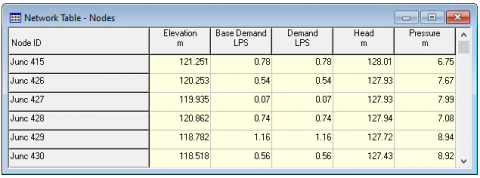Figure 2. Data set representing node properties

Table 1. Similarity matrix generation for the given dataset

 Similarity Matrix Node id 415 426 427 428 429 430 415 -12.611 -1.964 -4.284 -0.268 -11.265 -12.611 426 -1.964 -12.611 -0.645 -0.799 -4.590 -4.824 427 -4.284 -0.645 -12.611 -2.585 -4.652 -3.603 428 -0.268 -0.799 -2.585 -12.611 -8.187 -9.205 429 -11.265 -4.590 -4.652 -8.187 -12.611 -0.874 430 -12.611 -4.824 -3.603 -9.205 -0.874 -12.611

Table 2. Responsibility matrix for the given dataset
 Responsibility Matrix Node id 415 426 427 428 429 430 415 -2.320 1.789 -2.320 1.696 -9.301 -10.647 426 -4.216 -1.696 -2.897 -3.051 -6.842 -7.076 427 -3.639 2.958 -3.639 -1.940 -4.007 -2.958 428 0.531 1.789 -1.786 -3.485 -7.388 -8.406 429 -8.686 -2.011 -2.073 -5.608 -1.705 1.764 430 -10.091 -2.303 -1.083 -6.685 1.646 -1.705

Table 3. Availability matrix for the given dataset
 Availability Matrix Node id 415 426 427 428 429 430 415 0.531 -5.62E-11 -3.639 -3.485 -0.059 0 426 -1.789 6.536 -3.639 -1.789 -0.059 0 427 -1.789 -1.60E-10 1.06E-08 -1.789 -0.059 0 428 -2.320 -8.35E-10 -3.639 1.696 -0.059 0 429 -1.789 -5.62E-11 -3.639 -1.789 1.646 -1.705 430 -1.789 -5.62E-11 -3.639 -1.789 -1.705 1.764
4.3 Responsibility and availability matrix

Responsibility of column to row is the difference between similarity of column to row and the maximum corresponding to the remaining row similarities. The responsibility matrix (R) values are generated using Eq. (2), availability matrix (A) uses Eqns. (3) and (4) for off diagonal and diagonal elements respectively. Primarily, AVB(i,k) is initialized to zero. Iterations are then performed untill constant R and A values are obtained for the matrix using the relative equations. This is to perform numerical stabilization considering a damping factor within the specified range of 0.5 to 1. The RES matrix and AVB matrix are represented in Table 2 and Table 3.

4.4 Criterion matrix

Criterion matrix values are calculated as the sum of responsibility and availability as mentioned in Eq. (5). We calculate only diagonal elements because the off diagonal elements are not of use in the calculation to find exemplars. Hence, we make the diagonal elements of Criterion matrix equal to zero. The criterion matrix is represented in Table 4.

The positive diagonal values of the Criterion matrix are considered to form clusters with them as centres. The position of centres (ith row) is considered as centre index (i). Then, we compare all the centre indices with each row of the Similarity matrix. The centre index as column value corresponding to the maximum value in that particular row decides the cluster to which that particular node belongs. The exemplars generated from the given input are present in the Table 5.

From the above exempler values, nodes with id 415, 426,427 and 428 belong to same cluster with centre 426. 430 being the centre, another cluster is formed consisting node 429 and 430. The output for the suggested methodology is exhibited in Figure 3. Hence, the number of clusters formed is 2.

Table 4. Criterion matrix generation for the given dataset

 Criterion Matrix Node id 415 426 427 428 429 430 415 -1.789 0 0 0 0 0 426 0 4.841 0 0 0 0 427 0 0 -3.639 0 0 0 428 0 0 0 -1.789 0 0 429 0 0 0 0 -0.059 0 430 0 0 0 0 0 0.059

Table 5. Representation of exemplars of the given dataset
 Node-Ids Exemplers 415 2 426 2 427 2 428 2 429 6 430 6
4.5 AP-edge clustering

Consider the following WDN edge properties which are available in Figure 4. The data set consists of node length, diameter, roughness, flow, velocity, unit head loss and friction factor [12, 13] as properties for WDN edge table. Here, we consider six head records for understanding of the AP-WDN.

Generate similarity matrix, responsibility matrix, availability matrix and criterion matrix similarly as performed for the node data set. Exemplars are generated from the criterion matrix of edge dataset and the number of clusters formed for the considered six records from the data set is 2. The above mentioned matrices and exemplars are represented in Tables 6 to10 respectively.

## 3.png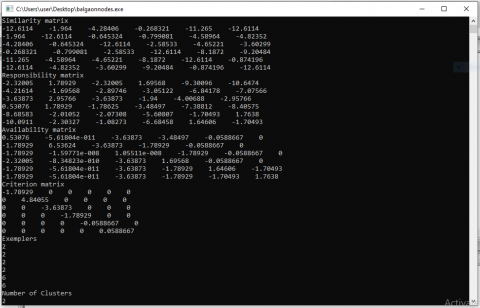Figure 3. Screenshot for output of node clustering

## 4.png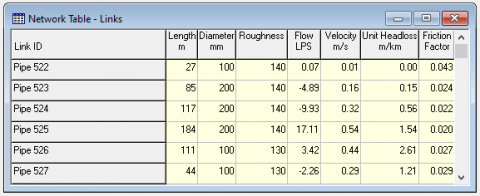Figure 4. Dataset representing Edge properties

Table 6. Similarity matrix for dataset of Edge properties

 Similarity Matrix Edge Id 522 523 524 525 526 527 522 -34894.2 -13387 -18193.2 -34894.2 -7143.78 -390.418 523 -13387 -34894.2 -1048.43 -9924.95 -10787.2 -11790.9 524 -18193.2 -1048.43 -34894.2 -4533.31 -10205.8 -15491.1 525 -34894.2 -9924.95 -4533.31 -34894.2 -15632.2 -29923.1 526 -7143.78 -10787.2 -10205.8 -15632.2 -34894.2 -4492.65 527 -390.418 -11790.9 -15491.1 -29923.1 -4492.65 -34894.2

Table 7. Responsibility matrix for dataset of Edge properties
 Responsibility Matrix Edge Id 522 523 524 525 526 527 522 -10396.8 -12996.6 -17802.8 -34503.7 -6753.36 10396.8 523 -12338.6 -9738.75 9738.75 -8876.53 -9738.75 -10742.5 524 -23398.6 -6253.86 -0.00048 -9738.74 -15411.3 -20696.5 525 -30360.8 -5391.64 6253.86 -6253.86 -11098.9 -25389.8 526 -2651.14 -6294.53 -5713.19 -11139.6 -6294.53 5713.19 527 -5713.18 -17113.7 -20813.8 -35245.8 -9815.41 -0.00186

Table 8. Availability matrix for dataset of Edge properties

 Availability Matrix Edge Id 522 523 524 525 526 527 522 3.74E-06 -9738.75 0 -6253.86 -6294.53 -8.78E-07 523 -10396.8 1.10E-06 -1.79E-07 -6253.86 -6294.53 -2.60E-09 524 -10396.8 -9738.75 15992.6 -6253.86 -6294.53 -2.60E-09 525 -10396.8 -9738.75 -2.91E-09 0 -6294.53 -2.60E-09 526 -10396.8 -9738.75 0 -6253.86 0 -7.39E-08 527 -10396.8 -9738.75 0 -6253.86 -6294.53 16109.9

Table 9. Criterion matrix for dataset of Edge properties
 Criterion Matrix Edge Id 522 523 524 525 526 527 522 -10396.8 0 0 0 0 0 523 0 -9738.75 0 0 0 0 524 0 0 15992.6 0 0 0 525 0 0 0 -6253.86 0 0 526 0 0 0 0 -6294.53 0 527 0 0 0 0 0 1610.9

Table 10. Exemplars for dataset of Edge properties
 Edge-Ids Exemplers 522 6 523 3 524 3 525 3 526 6 527 6
4.6 Algorithm: AP-WDN

Input: WDN dataset with node/edge properties, Number of data points (N)

Output: Clusters

Step 1: Read Number of data points (N) and the Input Data File

Step 2: Generate N x N Similarity matrix S.

Step 2.1: Calculate negative sum of squares of the Euclidian distance between the data points for off diagonal elements.

Step 2.2: Fill the diagonal elements with the minimum off diagonal value.

Step 3: Generate Responsibility matrix using

$\operatorname{RES}(\mathrm{i}, \mathrm{k})=\operatorname{SIM}(\mathrm{i}, \mathrm{k})-\operatorname{Max}_{\mathrm{k}^{\prime} \neq \mathrm{k}}\left\{\mathrm{AVB}\left(\mathrm{i}, \mathrm{k}^{\prime}\right)+\mathrm{SIM}\left(\mathrm{i}, \mathrm{k}^{\prime}\right)\right\}$

Step 4: Generate Availability matrix using

Step 4.1: For off diagonal elements

AVB(i,k)=Min(0,RES(k,k)+ $\left.\sum_{i^{\prime} \notin(i, k)} \operatorname{Max}\left(0, \operatorname{RES}\left(i^{\prime}, k\right)\right)\right)$

Step 4.2: For diagonal elements

$\mathrm{AVB}(\mathrm{k}, \mathrm{k})=\sum_{\mathrm{i}^{\prime} \notin(\mathrm{i}, \mathrm{k})} \operatorname{Max}\left(0, \mathrm{RES}\left(\mathrm{i}^{\prime}, \mathrm{k}\right)\right)$

Step 5: Iterate step 3 and 4 until constant values are obtained for RES matrix and AVB matrix.

Step 6: Generate CRT matrix by adding RES matrix and AVB matrix

CRT(i,k)=RES(i,k)+AVB(i,k)

Step 7: Generate exemplars using the positive diagonal values of the CRT matrix.

Step 8: Form clusters using the SIM matrix obtained and the exemplars obtained.

Step 9: Number of clusters is obtained as the output.

4.7 Time complexity

The proposed AP-WDN algorithm shows better performance as it implements vectorization function instead of nested implementation to reduce the number of iterations. Therefore, the time complexity is reduced to O(N2) from O(N2 I), where I represents iterations and N represents the count of data elements or nodes or edges  for any network.

5. Results

Real-world data-set for the Water Distribution Networkis located in Balgaon, Parvathipuram, Andhra Pradesh. It consists of 60 nodes and 73 edges which are considered for performing AP-WDN algorithm. Each node is having properties [12, 13] namely elevation in meters, base demand in litres per second (LPS), demand in LPS, head in meters and pressure in meters. The proposed technique is applied on the properties of 60 nodes. The WDN represented with node-ids are presented below in the Figure 5.

The nodes having same exemplar value belongs to the same cluster and the positive valued diagonal elements are considered as cluster centres. Here, six clusters are generated which are represented with different colors (blue, green, red, pink and purple). Each cluster centre is represented in yellow outlined with the color of the nodes in the corresponding cluster. The results of node clustering of AP-WDN are presented in Figure 6 and also in Table 11.

The clusters C1, C2, C3, C4, C5 and C6 formed by using AP-WDN are represented along with the node ids and node count in Table 11. The centres of each cluster are represented in blue.

## 5.pngFigure 5. Water distribution network for balgaon

## 6.pngFigure 6. Clusters formed by using AP-WDN

Table 11. Representation of clusters formed with nodes

 Cluster Node Ids Node Count C1 455, 457, 469, 470, 471, 472, 473, 474, 475, 476, 477, 480, 482, 483 14 C2 452, 453, 454, 456, 458, 459, 460, 464, 465, 466, 467, 468, 478, 479, 481, 484 16 C3 439, 441, 445, 447, 448, 450, 451, 461, 462, 463 10 C4 438, 440, 442, 443, 444, 446, 449 7 C5 427, 429, 430, 431, 432, 433, 434, 435, 436, 437 10 C6 415, 426, 428 3

## 7.png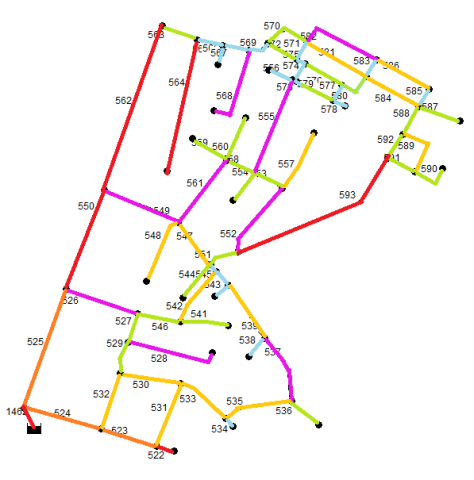Figure 7. Edge clusters along with outliers

Table 12. Edge clusters with cluster centres and outliers

 Clusters Edge-IDs Edge Count C1 526, 528, 537, 549, 552, 555, 561, 568, 582 9 C2 534, 538, 540, 543, 545, 556, 565, 566, 567, 569, 571, 573, 574, 575, 577, 578, 583, 585 18 C3 529, 536, 541, 544, 546, 551, 553, 554, 558, 559, 560, 563, 570, 572, 576, 579, 580, 587, 588, 590, 591, 592 23 C4 530, 531, 532, 533, 535, 539, 542, 547, 548, 557, 581, 584, 586, 589 14 Outliers 522, 1462, 593, 564, 562, 550, 525, 524, 523 9

The clustering process is performed on edge properties in the same way as it is done for node properties. The results obtained by AP-WDN for edges are represented in Figure 7 and Table 12. In Figure 7, each cluster is presented with different color and the details present in this Figure 7 are explored in Table 12 by specifying the clusters C1, C2, C3 and C4 with edge-ids and count. Centre of each cluster is represented with pink color.

Using AP-WDN on edge properties, we are able to identify outliers which are represented in red in Figure 7 and in Table 12.

5.1 Result analysis

We consider the same network WDN shown in Figure 5 to perform K-Means clustering using Weka 3.6.9 as shown in Figure 8. The number of clusters are six. They are C0, C1, C2, C3, C4 and C5 and the results obtained are represented below in Table 13. The performance analysis of AP and K-Means clustering on WDN is represented in Figure 9 and Figure 10.

## 8.png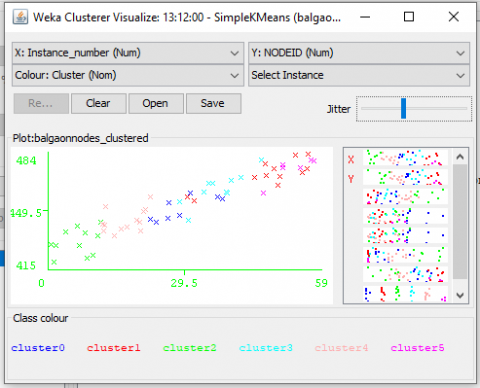Figure 8. WDN Clusters representation using K-Means

Table 13. Clusters formed using K-Means on WDN node properties

 Clusters Nodes Nodes Count C0 448, 450, 451, 452, 453, 458, 461, 462 8 C1 455, 457, 470, 471, 472, 473, 474, 475, 479, 480, 481, 483 12 C2 415, 426, 427, 428,429, 430, 431, 432, 433, 434, 435 11 C3 454, 456, 459, 460, 463, 464, 465, 466, 467, 468 10 C4 436, 437, 438, 439, 440, 441, 442, 443, 444, 445, 446, 447, 449 13 C5 469, 476, 477, 478, 482, 484 6

## 9.pngFigure 9. AP vs K-means clustering on WDN

## 10.png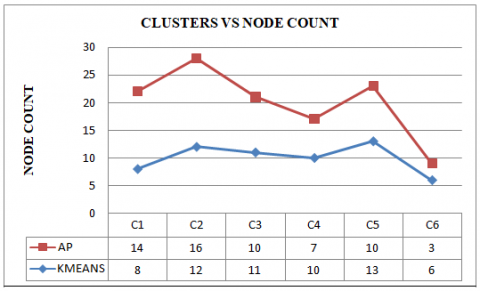Figure 10. Clusters vs node count in each cluster

6. Conclusion

The paper proposes AP-WDN algorithm, which performs clustering using affinity propagation on node and edge properties of a real-world infra structure network, water distribution network. Most real-world networks are complex. They have both structural and behavioural properties. Hence we have consider both edge and node properties of the water distribution network. AP-WDN shows better performance as it implements vectorization function instead of nested implementation to reduce the number of iterations. Therefore, the time complexity is reduced to O(N2) from O(N2 I). The time complexity relatively decreases for sparse networks. AP-WDN is more efficient than K-Means but time complexity is nearly equal. AP-WDN is having an advantage over K-Means as the necessity of specifying the count of clusters is absent. Also, the cluster centres are the nodes or edges themselves (data elements). Using AP-WDN we can a well find the outliers. AP-WDN results in less than $\surd$N clusters and also reduced time complexity. If we consider large networks and adopt divide and conquer mechanism the time complexity will be further reduced.

References

 Frey, J.B., Delbert, D. (2007). Clustering by passing messages between data points. Science, 315: 972-976. http://doi.org/10.1126/science.1136800

 Xia, D., Wu, F., Zhang, X.Q., Zhuang, Y.T. (2008). Local and global approaches of affinity propagation clustering for large scale data. Journal of Zhejiang University-SCIENCE A, 9: 1373-1381. http://doi.org/10.1631/jzus.A0720058

 Shang, F.H., Jiao, L.C., Shi, J.R., Wang, F., Gong, M.G. (2012). Fast affinity propagation clustering: A multilevel approach. Pattern Recognition, 45(1): 474-486. https://doi.org/10.1016/j.patcog.2011.04.032

 Zhao, X.L., Xu, W.X. (2015). An extended affinity propagation clustering method based on different data density types. Computational Intelligence and Neuroscience, 2015: 1-8. http://doi.org/10.1155/2015/828057

 Xie, Y.F., Wang, X., Zhang, L., Yu, G.X. (2019). Affinity propagation clustering using centroid-deviation-distance based similarity. HBAI 2019: Human Brain and Artificial Intelligence, 286-299. http://doi.org/10.1007/978-981-15-1398-5_21

 Bateni, M., Behnezhad, S., Derakhshan, M., Hajiaghayi, M., Kiveris, R., Lattanzi, S., Mirrokni, V.S. (2017). Affinity clustering: Hierarchical clustering at scale. In Proceedings of the Advances in Neural Information Processing Systems 30: Annual Conference on Neural Information Processing Systems, Long Beach, CA, USA, pp. 6867-6877.

 Wang, L.M., Zheng, K.Y., Tao, X., Han, X.M. (2018). Affinity propagation clustering algorithm based on large-scale data-set. International Journal of Computers and Applications, 40(3): 1-6. http://doi.org/10.1080/1206212X.2018.1425184

 Yang, J., Wang, Y., Sowmya, A., Zhang, B., Xu, J., Li, Z.D. (2010). Affinity propagation feature clustering with application to vehicle detection and tracking in road traffic surveillance. Advanced Video and Signal Based Surveillance, Boston, MA, USA, pp. 414-419. http://doi.org/10.1109/AVSS.2010.40

 Matsushita, T., Shiokawa, H., Kitagawa, H. (2018). C-AP: Cell-based algorithm for efficient affinity propagation. In Proceedings of the 20th International Conference on Information Integration and Web-based Applications & Services, WAS2018, pp. 156-163. http://doi.org/10.1145/3282373.3282377

 Moiane, A.F., Machado, Á.M.L. (2018). Evaluation of the clustering performance of affinity propagation algorithm considering the influence of preference parameter and damping factor. Boletim de Ciências Geodésicas, 24(4): 426-441. http://doi.org/10.1590/s1982-21702018000400027

 Jeong, B., Lee, J., Cho, H. (2010). Improving memory-based collaborative filtering via similarity updating and prediction modulation. Information Sciences, 180(5): 602-612. http://doi.org/10.1016/j.ins.2009.10.016

 Santhi, S.V.S., Padmaja, P. (2016). An efficient and refined minimum spanning tree approach for large water distribution system. International Journal of Decision Support System (IJDSS), 2(1): 181-206. http://doi.org/10.1504/IJDSS.2016.081777

 Santhi, S.V.S., Padmaja, P. (2016). Large graph mining approach for cluster analysis to identify critical components within the water distribution system. International Journal of Computer Science and Information Technologies (IJCSIT), 7(1): 384-391.